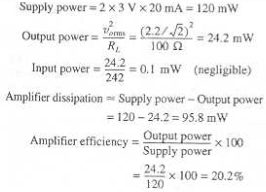Courses

# Test: Amplifiers

## 10 Questions MCQ Test Analog Electronics | Test: Amplifiers

Description
This mock test of Test: Amplifiers for Electrical Engineering (EE) helps you for every Electrical Engineering (EE) entrance exam. This contains 10 Multiple Choice Questions for Electrical Engineering (EE) Test: Amplifiers (mcq) to study with solutions a complete question bank. The solved questions answers in this Test: Amplifiers quiz give you a good mix of easy questions and tough questions. Electrical Engineering (EE) students definitely take this Test: Amplifiers exercise for a better result in the exam. You can find other Test: Amplifiers extra questions, long questions & short questions for Electrical Engineering (EE) on EduRev as well by searching above.
QUESTION: 1

### An amplifier operating from ±3V provide a 2.2V peak sine wave across a 100 ohm load when provided with a 0.2V peak sine wave as an input from which 1.0mA current is drawn. The average current in each supply is measured to be 20mA. What is the amplifier efficiency?

Solution:QUESTION: 2

### In order to prevent distortion in the output signal after amplification, the input signal must be

Solution:

Higher than the positive saturation and lower than the negative saturation level of the amplifier are the desired characteristics in order to prevent distortion.

QUESTION: 3

### The voltage gain of the amplifier is 8 and the current gain is 7. The power gain of the amplifier is

Solution:

The power gain is given by 10 log (7 X 8) db.

QUESTION: 4

Statement 1: Voltage gain of -5 means that the output voltage has been attenuated.Statement 2: Voltage gain of -5db means that the output voltage has been attenuated.

Solution:

A negative voltage gain means that a phase difference of 1800 has been introduced in the output waveform when compared to the input waveform. A voltage gain of -5db means that the signal has been attenuated.

QUESTION: 5

Which of the following isn’t true?

Solution:

For an ideal transformer the power input is always equal to the power output. In real conditions there is slight loss of power when transferring the power from an input source to an output source. Amplifiers only provide power gain.

QUESTION: 6

Symmetrically saturated amplifiers operating in clipping mode can be used to convert a sine wave to a

Solution:

Clipping circuits with low peak values of the output signals are used to generate pseudo square waves if the input signal is very large as compared to the output signal.

QUESTION: 7

What is meant by stability of the an amplified signal?

Solution:

The ability of the amplifier to prevent self oscillation is a measure of its stability

QUESTION: 8

If Av, Ai and Ap represents the voltage gain, current gain and power gain ratio of an amplifier which of the below is not the correct expression for the corresponding values in decibel?

Solution:

Power gain is given by 10 log Ap db.

QUESTION: 9

An amplifier has a voltage gain of 100 V/V and a current gain of 1000A/A. the value of the power gain decibel is

Solution:

Power gain in db is given by 10 log (100 X 1000) db.

QUESTION: 10

The units of voltage gain is

Solution:

Voltage gain (Vo) = output voltage/input voltage (Vi). It is also expresses as 20 log (Vo/Vi) db.